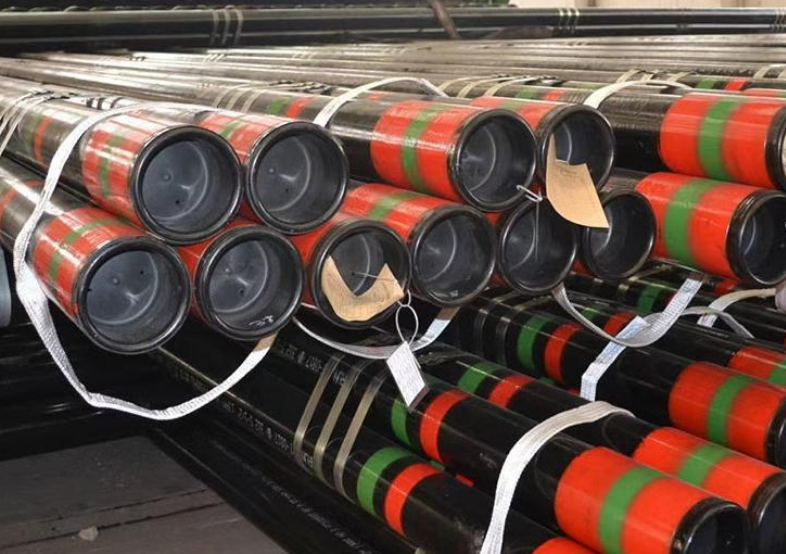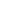Home  >  Hot News  >  Current News

# Oil Casing Weight Calculation Method and Influencing Factors

Date:2023-11-16    keywords: oil casing weight calculation, casing influencing factors, casing pipe
Calculation method of oil casing pipe weight:

The weight of the casing can be calculated by the following formula:
Weight (kg) = length (m) × diameter (mm) × wall thickness (mm) × material density (kg/m³) × π/4

Among them, the length is the actual length of the casing, the diameter is the average of the inner diameter and the outer diameter, the wall thickness is the outer diameter minus half of the inner diameter, the material density can generally be found in the material manual, and π is pi.

For example, a casing with a diameter of 50mm, a wall thickness of 4mm, and a length of 3m has a material density of 7800kg/m³, and its weight is calculated as follows:
Weight (kg) = 3m × (50mm+54mm)/2 × (54mm-50mm)/2 × 7800kg/m³ × π/4

= 3m × 52mm × 2mm × 7800kg/m³ × 3.14/4 = 48.7kgFactors affecting oil casing pipe weight calculation:

1. Effect of casing material on weight
Different materials have different densities and have different effects on weight. For example, the weight of steel and copper casings of the same size will be very different. In engineering design, casing materials should be carefully selected to meet engineering needs while controlling weight.

2. Influence of casing size and quantity
The size and number of casings also have a significant impact on weight. If it is a large diameter or long length casing, its weight will be correspondingly larger. At the same time, you need to pay attention to the number of casings, because the weight of the casings will also increase with the increase in number. In engineering design, the size and quantity of casing should be determined according to actual conditions to control weight.

3. Guarantee of calculation accuracy
The weight calculation formula of casing is a theoretical calculation, and different calculation accuracy has different effects on the results. Therefore, the calculation accuracy should be ensured as much as possible and the error should be reduced during calculation to ensure the accuracy of the calculation results.

In short, the weight calculation of casing needs to consider many factors, including the size, quantity, material, calculation accuracy, etc. of the casing. In actual engineering design, calculations should be made based on actual conditions and the influence of various factors should be paid attention to to ensure that the weight of the casing is controlled within a reasonable range.